### Home > CALC > Chapter 6 > Lesson 6.4.2 > Problem6-141

6-141.
1. Find and compare the average value of each of these functions over one complete cycle. Homework Help ✎

1. f(t) = sin t

2.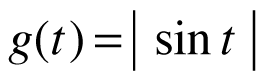3. k(t) = (sin t)2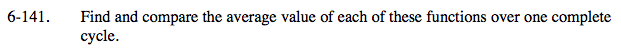For each of the functions below, we are given f(x) and we want to find the average value of f(x).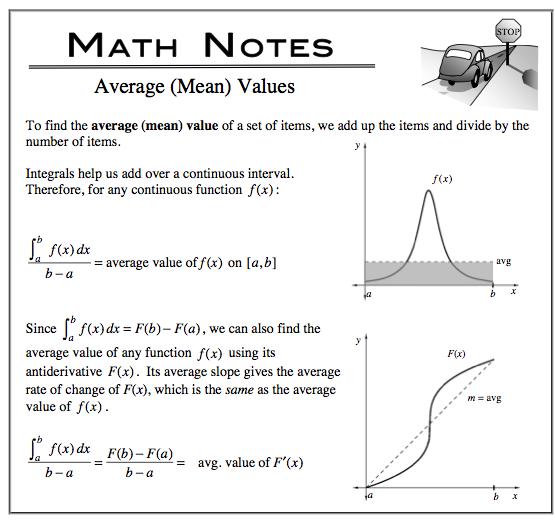y = sinx has a period of 2π.
So choose a and b values such that Δx = 2π.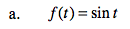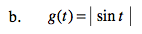Compare the periods of y = |sinx| and y = sinx.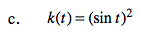$\text{cos}(t-\frac{\pi }{2})=\text{sin}t, (\text{sin}t)^{2}=\text{cos}^{2}(t-\frac{\pi }{2})=\text{cos}^{2}t$

Did you realize that since

$\text{So}\int_{0}^{2\pi}\text{sin}^{2}(x)dx=\frac{1}{2}\int_{0}^{2\pi}\text{sin}^{2}(x)+\text{cos}^{2}(x)dx$

$\frac{\int_{0}^{2\pi }g(x)dx}{2\pi - 0}=\frac{1}{2}$